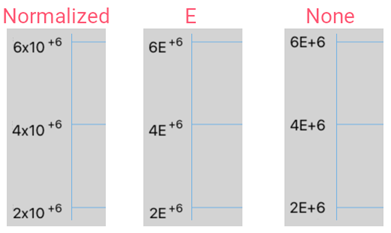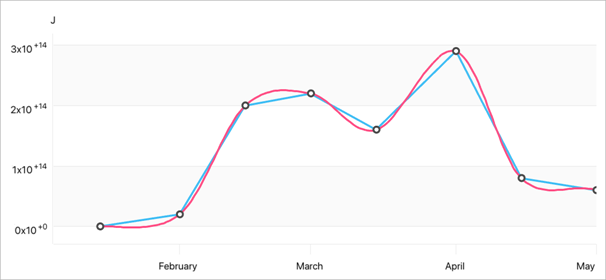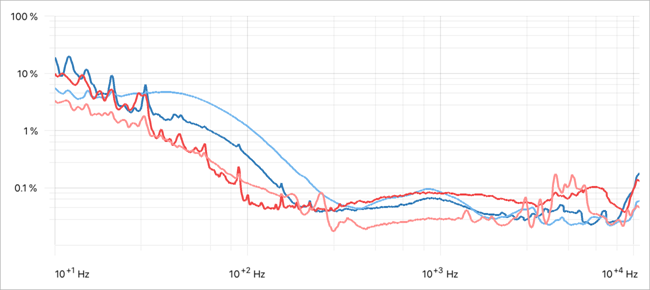# AxisLabelScientificNotation Class

Converts axis label numbers to scientific notation.

Namespace: DevExpress.Maui.Charts

Assembly: DevExpress.Maui.Charts.dll

NuGet Package: DevExpress.Maui.Charts

## Declaration

``````public class AxisLabelScientificNotation :
AxisLabelNotationBase``````

## Remarks

If axis labels display very large or very small numbers that take up a large amount of space, you can use scientific or engineering notation to display these numbers.

In scientific notation, a number is displayed as m×10ⁿ, where the coefficient m is any real number, and the exponent n is an integer. The following scientific notations are supported:

• Normalized - the exponent n is selected so that the coefficient m is at least 1 but less than 10.
• E - ×10 is replaced with E.
• Logarithmic - bases other than 10 can be used (for example, m×2ⁿ).

### Normalized and E Notations

1. Assign an AxisLabelScientificNotation object to the LabelValueNotation property of a numeric axis object.
2. Set the AxisLabel.TextFormat property to “#E+0”.
3. Set the AxisLabelScientificNotation.ScientificNotation property to the Normalized (default) or E value.This example shows how to convert numbers that Y-axis labels display to normalized notation.``````<dxc:ChartView>
<dxc:ChartView.Series>
<!-- Series data. -->
</dxc:ChartView.Series>

<dxc:ChartView.AxisY>
<dxc:NumericAxisY>
<dxc:NumericAxisY.LabelValueNotation>
<dxc:AxisLabelScientificNotation/>
</dxc:NumericAxisY.LabelValueNotation>
<dxc:NumericAxisY.Label>
<dxc:AxisLabel TextFormat="#E+0"/>
</dxc:NumericAxisY.Label>
<!-- Other settings of the Y-axis. -->
</dxc:NumericAxisY>
</dxc:ChartView.AxisY>

<dxc:ChartView.AxisX>
<!-- The X-axis settings. -->
</dxc:ChartView.AxisX>
</dxc:ChartView>
``````

### Logarithmic Notation

1. Assign an AxisLabelScientificNotation object to the LabelValueNotation property of a numeric axis object.
2. Set the AxisLabelScientificNotation.ScientificNotation property to Logarithmic.

This example shows how to apply logarithmic notation to labels of an X-axis that uses the logarithmic scale to display numeric values. This notation uses powers of a base that the AxisLogarithmicOptions.Base property defines (10, in this example).``````<dxc:ChartView >
<dxc:ChartView.Series>
<!-- Series data. -->
</dxc:ChartView.Series>

<dxc:ChartView.AxisX>
<dxc:NumericAxisX>
<dxc:NumericAxisX.LogarithmicOptions>
<dxc:AxisLogarithmicOptions Enabled="True" Base="10"/>
</dxc:NumericAxisX.LogarithmicOptions>
<dxc:NumericAxisX.LabelValueNotation>
<dxc:AxisLabelScientificNotation ScientificNotation="Logarithmic"/>
</dxc:NumericAxisX.LabelValueNotation>
<dxc:NumericAxisX.Label>
<dxc:AxisLabel TextFormat="# Hz"/>
</dxc:NumericAxisX.Label>
<!-- Other settings of the X-axis. -->
</dxc:NumericAxisX>
</dxc:ChartView.AxisX>

<dxc:ChartView.AxisY>
<dxc:NumericAxisY>
<dxc:NumericAxisY.LogarithmicOptions>
<dxc:AxisLogarithmicOptions Enabled="True" Base="10"/>
</dxc:NumericAxisY.LogarithmicOptions>
<dxc:NumericAxisY.Range>
<dxc:NumericRange SideMargin="0" Min="0.01" Max="100"/>
</dxc:NumericAxisY.Range>
<!-- Other settings of the Y-axis -->
</dxc:NumericAxisY>
</dxc:ChartView.AxisY>
</dxc:ChartView>
``````